## 2.2Velocity

Like force, velocity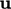is a vector with direction and magnitude, with SI units of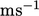. Using the vector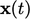to denote the position of a particle of ﬂuid, its velocity is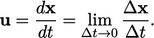(2.4)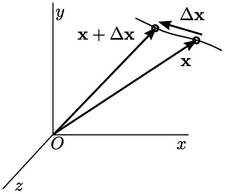### Vector ﬁelds

Whilecan be used to denote a single velocity with magnitude and direction, it can also denote a vector ﬁeld of velocity which varies from point to point across a spatial domain. A vector is represented by 3 numbers, relating to the co-ordinate system being used, e.g.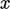,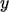,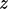, in the Cartesian system.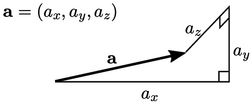While the magnitude and direction of a vector is ﬁxed, it is not invariant since the 3 values depend on the co-ordinate system used. We represent a vector without reference to the co-ordinate system by bold text, e.g.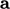” (compared to scalar “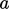”).

### Basic vector algebra

Addition and subtraction of 2 vectors is performed by operating on respective components. Subtraction of two vectorsand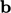is performed by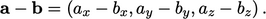Multiplication of any vectorby a scalar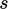is performed by multiplying all the components by the scalar, e.g.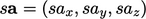Addition and multiplication are commutative, i.e. variables can be in any order (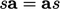). Subtraction is not commutative. Products between scalars and vectors are distributive, i.e.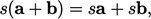and with additional scalar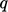,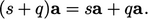Division between a vector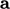and a scalar is only relevant when the scalar is the second argument of the operation, i.e.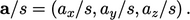Notes on CFD: General Principles - 2.2 Velocity